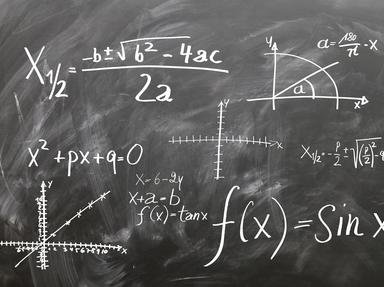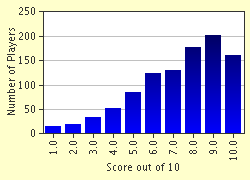# Simple Mixed Math Trivia Quiz

### Test your knowledge on some simple math word problems.

A multiple-choice quiz by Legolas6000. Estimated time: 4 mins.

Author
Legolas6000
Time
4 mins
Type
Multiple Choice
Quiz #
154,000
Updated
Dec 03 21
# Qns
10
Difficulty
Average
Avg Score
7 / 10
Plays
8381
Last 3 plays: Guest 24 (9/10), Guest 97 (2/10), Guest 72 (6/10).
This quiz has 2 formats: you can play it as a or as shown below.
Scroll down to the bottom for the answer key.
1. Lindsay is building a swimming pool. On the blueprint for the pool the scale is 3in. to every 5ft. If the length of the pool is 9in. on the blueprint how many feet is the actual length of the pool? Hint

17ft.
27ft.
25ft.
15ft.

#### NEXT>

2. Christopher ordered an eighteen slice pizza from Crazy Joe's Pizzeria. For dinner, Chris ate 3 slices. In simplest form, what fraction of the pie did he eat? Hint

2/16
1/4
1/18
1/6

#### NEXT>

3. Robert is 2 years older than Vivian. The sum of their ages is 106. How old is Vivian? Hint

54
52
45
53

#### NEXT>

4. Jessica bought a dress that was originally \$68.00. The sales tax is 8.5% but she had a 20% off coupon. How much did Jessica pay for the dress? Hint

\$61.37
\$59.03
\$70.84
\$57.42

#### NEXT>

5. Brian is eating a bag of Skittles. In the bag there are 5 red, 2 green, and 4 purple Skittles. What is the probability that Brian will pick a red Skittle, eat it, and then pick a green Skittle? Hint

1/12
1/11
15/110
10/121

#### NEXT>

6. Erin reads an average of 20 books per year. She read 23, 17, 20, and 19 books in four different years. How many books did Erin read in the 5th year to make it an average of exactly 20.0 per year? Hint

17
20
21
19

#### NEXT>

7. Katelyn owns a candy shop. The ratio of Milky Way bars to Snickers bars is 2 to 3. The ratio of Starbursts to Milky Way bars is 1 to 2. If Katelyn has 51 Snickers bars how many Starbursts does she have?

#### NEXT>

8. Michael ate a pound of steak for dinner. Gina ate 3 eighths of a pound and Jimmy ate 19 twenty-fourths of a pound. How much steak did they eat in total? Hint

2 pounds
2 and 17/24 pounds
2 and 15/32 pounds
2 and 1/6 pounds

#### NEXT>

9. Kelly, Orlando, and James received 65 Christmas presents in total. James received 7 more presents than Kelly and Orlando received 13 more presents than Kelly. How many presents did Orlando receive?

#### NEXT>

10. Paul took a science test in school. If he got 23 out of 27 questions right what percentage did he score? Please round to the nearest percent. Hint

92%
82%
77%
85%

 (Optional) Create a Free FunTrivia ID to save the points you are about to earn:Select a User ID:Choose a Password:Your Email:

Quiz Answer Key and Fun Facts
1. Lindsay is building a swimming pool. On the blueprint for the pool the scale is 3in. to every 5ft. If the length of the pool is 9in. on the blueprint how many feet is the actual length of the pool?

The easiest way to find the answer is to use a proportion. The inches always stay on the top and the feet stay on the bottom. 3in. over 5ft. = 9in. over xft. After setting up the proportion you take the cross products. 5*9 = 3x. (3x = 45). Then you divide each side by 3 to get 15ft. Don't forget the units!
2. Christopher ordered an eighteen slice pizza from Crazy Joe's Pizzeria. For dinner, Chris ate 3 slices. In simplest form, what fraction of the pie did he eat?

Chris ate 3/18 of the pizza but to get it in simplest form you have to divide the numerator and denominator by a common factor which in this case is 3. Therefore, Chris ate 1/6 of the pie.
3. Robert is 2 years older than Vivian. The sum of their ages is 106. How old is Vivian?

You can solve this problem using the equation x+(x+2) = 106. Let x = Vivian's age. First you distribute. x+x+2 = 106. Then you combine like terms. 2x+2 = 106. Next you subtract 2 from both sides. 2x = 104. Finally you divide each side by 2 to get 52 years.
4. Jessica bought a dress that was originally \$68.00. The sales tax is 8.5% but she had a 20% off coupon. How much did Jessica pay for the dress?

First you subtract the discount and then you add the tax. 20% of 68 is 13.60. 13.60 taken away from 68 is 54.40. 8.5% of 54.40 is 4.624. 4.624 added to 54.40 is 59.024. If you round to the tenths place it comes to \$59.02. You must always round up when dealing with money in order not to lose any money so the answer is 59.03.
5. Brian is eating a bag of Skittles. In the bag there are 5 red, 2 green, and 4 purple Skittles. What is the probability that Brian will pick a red Skittle, eat it, and then pick a green Skittle?

The probability of picking a red Skittle is 5/11. The probability of picking a green Skittle is 2/11 but since he ate a red Skittle there are no longer 11 Skittles. The probability of picking a green Skittle would therefore be 2/10 or 1/5. When you multiply 5/11 times 1/5 it comes out to 5/55. The all you need to do is simplify your answer to 1/11.
6. Erin reads an average of 20 books per year. She read 23, 17, 20, and 19 books in four different years. How many books did Erin read in the 5th year to make it an average of exactly 20.0 per year?

Using the equation 23+17+20+19+x=20*5 you can figure out how many books she needed to read in order to keep the average 20. After you combine like terms the equation is 79+x = 100. 100 minus 79 is 21. If Erin reads 21 books her average will be 20 books per year.
7. Katelyn owns a candy shop. The ratio of Milky Way bars to Snickers bars is 2 to 3. The ratio of Starbursts to Milky Way bars is 1 to 2. If Katelyn has 51 Snickers bars how many Starbursts does she have?

First you set up a proportion comparing Milky Ways to Starbursts. 2 over 3 = x over 51. x = 34. You have 34 Milky Way bars. Then you can set up the proportion comparing Starbursts to Milky Ways. 1 over 2 = x over 34. x = 17. There are 17 Starbursts.
8. Michael ate a pound of steak for dinner. Gina ate 3 eighths of a pound and Jimmy ate 19 twenty-fourths of a pound. How much steak did they eat in total?

First you need to find a common denominator for the fractions. The easiest common denominator would be 24. Then you add up the fractions. 1 + 9/24 + 19/24 = 13/6. Then you need to change the improper fraction to a mixed number. 13/6 = 2 and 1/6.
9. Kelly, Orlando, and James received 65 Christmas presents in total. James received 7 more presents than Kelly and Orlando received 13 more presents than Kelly. How many presents did Orlando receive?

You can figure out how many presents Kelly received by using the equation x+x+7+x+13 = 65. First you combine like terms. 3x+20 = 65. then you subtarct 20 on both sides. 3x = 45. Lastly you divide both sides by 3. x = 15. Kelly received 15 presents. To find the amount of presents Orlando received you have to add 13 to the amount of presents Kelly received. 15+13 = 28. Orlando received 28 presents.
10. Paul took a science test in school. If he got 23 out of 27 questions right what percentage did he score? Please round to the nearest percent.

Source: Author Legolas6000

This quiz was reviewed by FunTrivia editor crisw before going online.
Any errors found in FunTrivia content are routinely corrected through our feedback system.
Most Recent Scores
Jan 20 2023 : Guest 24: 9/10
Jan 19 2023 : Guest 97: 2/10
Jan 19 2023 : Guest 72: 6/10
Jan 09 2023 : Guest 93: 4/10
Dec 28 2022 : Guest 24: 8/10
Dec 28 2022 : Guest 118: 10/10
Dec 16 2022 : Guest 46: 5/10
Dec 13 2022 : Guest 65: 2/10
Dec 08 2022 : Guest 69: 5/10

Score Distribution